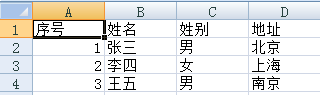# Python如何使用Excel文件<pre class="brush:php;toolbar:false">#!/usr/bin/python3 # -*- coding:utf-8 -*-   # 导入模块 import win32com.client   # 打开Excel Application = win32com.client.Dispatch("Excel.Application") # 或者使用下面的方法，使用启动独立的进程： # Application = win32com.client.DispatchEx("Excel.Application")   # 后台运行，显示程序界面，不警告 Application.Visible = 1  # 调试阶段建议打开 Application.DisplayAlerts = 0   # 新建一个文档 Workbook = Application.Workbooks.Add()   # 打开一个文档 Workbook = Application.Workbooks.Open("F:\\test.xlsx") # 根据工作表名 Base = Workbook.Worksheets("Sheet1") # 根据工作表顺序 # Base = Workbook.Worksheets(1)   # 接受当前工作表 # Base = Workbook.ActiveSheet   # 添加内容: 0.0, 0.5, 1.0 Base.Cells(1, 1).Value = 'Values' Base.Cells(1, 2).Value = 0.0 Base.Cells(1, 3).Value = 0.5 Base.Cells(1, 4).Value = 1.0   Workbook.SaveAs("F:\\test.xlsx")   # 关闭文档 Workbook.Close()   # 退出Excel Application.Quit()</pre>

<pre class="brush:php;toolbar:false">#!/usr/bin/python3 # -*- coding:utf-8 -*-   # 导入模块 import win32com.client   # 打开Excel Application = win32com.client.Dispatch("Excel.Application") # 或者使用下面的方法，使用启动独立的进程： # Application = win32com.client.DispatchEx("Excel.Application")   # 后台运行，显示程序界面，不警告 Application.Visible = 1  # 调试阶段建设打开 Application.DisplayAlerts = 0   # 打开一个文档 Workbook = Application.Workbooks.Open("F:\\test.xlsx") Base = Workbook.Worksheets(1)   # 接受当前工作表 Base = Workbook.ActiveSheet   #列数 ncols = 0 while True:     cell_value = Base.Cells(1, ncols   1).Value     if cell_value:         ncols  = 1     else:         break   # 行数 nrows = 0 while True:     cell_value = Base.Cells(nrows   2, 1).Value  # 第一行表头     if cell_value:         for col in range(ncols):             cell_key = Base.Cells(1, col   1).Value             cell_value = Base.Cells(nrows   2, col   1).Value             print(cell_key, "=>", cell_value, end = "\t")         print("")         nrows  = 1     else:         break   # 关闭文档 Workbook.Close()   # 退出Excel Application.Quit()</pre><pre class="brush:php;toolbar:false">序号 => 1.0    姓名 => 张三 姓别 => 男  地址 => 北京 序号 => 2.0    姓名 => 李四 姓别 => 女  地址 => 上海 序号 => 3.0    姓名 => 王五 姓别 => 男  地址 => 南京</pre>

[]
~ ~